Importance: Medium ✭✭
 Author(s): Mohar, Bojan
 Subject: Graph Theory » Infinite Graphs
 Keywords: hamiltonian infinite graph uniquely hamiltonian
 Posted by: Robert Samal on: July 24th, 2007
Problem   Are there any uniquely hamiltonian locally finite 1-ended graphs which are regular of degree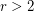?

(Originally appeared as [M].)

Letbe a locally finite infinite graph and let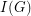be the set of ends of~. The Freudenthal compactification ofis the topological space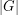which is obtained from the usual topological space of the graph, when viewed as a 1-dimensional cell complex, by adding all points ofand setting, for each end, the basic set of neighborhoods ofto consist of sets of the form, whereranges over the finite subsets of,is the component of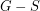containing all rays in, the set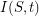contains all ends inhaving rays in, andis the union of half-edges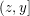, one for every edgejoiningand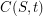. We define a hamilton circle inas a homeomorphic image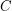of the unit circle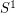intosuch that every vertex (and hence every end) ofappears in. More details about these notions can be found in [D].

A graph(finite or infinite) is said to be uniquely hamiltonian if it contains precisely one hamilton circle.

For finite graphs, the celebrated Sheehan's conjecture states that there are no-regular uniquely hamiltonian graphs for; this is known for all oddand even. For infinite graphs this is false even for odd(e.g. for the two-way infinite ladder), but each of the known counterexamples has at least 2 ends, leading to the problem stated.

Another way to extend Sheehan's conjecture to infinite graphs is to define degree of an endto be the maximal number of disjoint rays inand ask the following:

Problem   Are there any uniquely hamiltonian locally finite graphs where every vertex and every end has the same degree?

Bibliography

[D] R. Diestel, Graph Theory, Third Edition, Springer, 2005.

*[M] Bojan Mohar, Problem of the Month

* indicates original appearance(s) of problem.